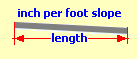Spike's Calculators

# Elevation Grade from Inches per FootThis calculator will calculate the drop or rise in inches, the difference in elevation, from a slope expressed in inches per foot.

##### Example calculation
```a 40 foot length of pipe requires a 1/8" drop per foot
40 * 1/8 = 40/8 = 5```
The required drop from start of the pipe to the end is 5"

### Elevation Grade / Slope of Inches per Foot

Length ft in
Slope per Foot in n
d
Fraction Precision #

#### Results:

 Difference in Height in

#### Calculation

1. length in feet & inches
2. required slope inches per foot
3. fraction precision preset @16

#### Result

1. required drop in inches